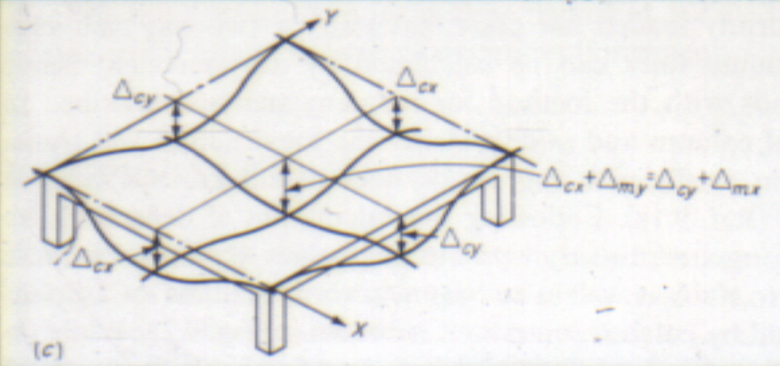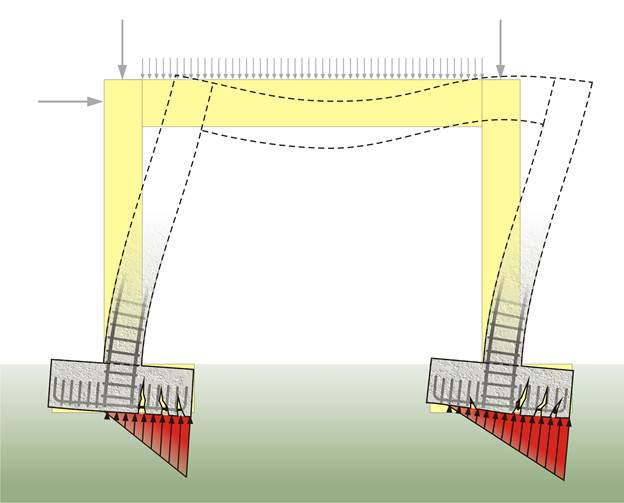# السلام عليكم وكل عام وانتم بخير

#### علاء سعود عبيدات

##### عضو جديد
اريد شرح عن deflection

### مواضيع مماثلة

#### د.م يوسف حميضة

##### إستشاري الهندسة المدنية
إستشاري
اريد شرح عن deflection

حساب الانتقال والسهم

deflection for various loads and supportsBeam deflection for various loads and supports

Beams can vary greatly in their geometry and composition. For instance, a beam may be straight or curved. It may be of constant cross section, or it may taper. It may be made entirely of the same material (homogeneous), or it may be composed of different materials (composite). Some of these things make analysis difficult, but many engineering applications involve cases that are not so complicated. Analysis is simplified if:
-The beam is originally straight, and any taper is slight-The beam experiences only linear elastic deformation-The beam is slender (its length to height ratio is greater than 10)-Only small deflections are considered (max deflection less than 1/10 the span)
In this case, the equation governing the beam's deflection () can be approximated as:where the second derivative of its deflected shape with respect tois interpreted as its curvature,is the Young's modulus,is the area moment of inertia of the cross-section, andis the internal bending moment in the beam.
If, in addition, the beam is not tapered and is homogeneous, and is acted upon by a distributed load, the above expression can be written as:This equation can be solved for a variety of loading and boundary conditions. A number of simple examples are shown below. The formulas expressed are approximations developed for long, slender, homogeneous, prismatic beams with small deflections, and linear elastic properties. Under these restrictions, the approximations should give results within 5% of the actual deflection.
Cantilever beams

Cantilever beams have one end fixed, so that the slope and deflection at that end must be zero.

End-loaded cantilever beams

The elastic deflectionand
angle of deflection(in
radians) at the free end in the example image: A (weightless) cantilever beam, with an end load, can be calculated (at the free end B) using:[SUP][/SUP]where= Force acting on the tip of the beam= Length of the beam (span)= Modulus of elasticity= Area moment of inertia of the beam's cross section
Note that if the span doubles, the deflection increases eightfold. The deflection at any point,, along the span of an end loaded cantilevered beam can be calculated using:[SUP][/SUP]Note that at(the end of the beam), theandequations are identical to theandequations above.

Uniformly-loaded cantilever beams

The deflection, at the free end B, of a cantilevered beam under a uniform load is given by:[SUP][/SUP]where= Uniform load on the beam (force per unit length)= Length of the beam= Modulus of elasticity= Area moment of inertia of cross section
The deflection at any point,, along the span of a uniformly loaded cantilevered beam can be calculated using:[SUP][/SUP]Simply-supported beam

Simply-supported beams have supports under their ends which allow rotation, but not deflection.

Center-loaded simple beams

The elastic deflection (at the midpoint C) of a beam, loaded at its center, supported by two simple supports is given by:[SUP][/SUP]where= Force acting on the center of the beam= Length of the beam between the supports= Modulus of elasticity= Area moment of inertia of cross section
The deflection at any point,, along the span of a center loaded simply supported beam can be calculated using:[SUP][/SUP]

forOff-center-loaded simple beams

The maximum elastic deflection on a beam supported by two simple supports, loaded at a distancefrom the closest support, is given by:[SUP][/SUP]where= Force acting on the beam= Length of the beam between the supports= Modulus of elasticity= Area moment of inertia of cross section= Distance from the load to the closest support (i.e.)
This maximum deflection occurs at a distancefrom the closest support and is given by:[SUP][/SUP]Uniformly-loaded simple beams

The elastic deflection (at the midpoint C) on a beam supported by two simple supports, under a uniform load (as pictured) is given by:[SUP][/SUP]where= Uniform load on the beam (force per unit length)= Length of the beam= Modulus of elasticity= Area moment of inertia of cross section
The deflection at any point,, along the span of a uniformly loaded simply supported beam can be calculated using:[SUP][/SUP]التعديل الأخير:

#### Mohamed_Osman09

##### عضو جديد
ماشاء الله ما قصر معاك المهندس شرح وافي ..